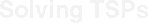........World TSPNational TSPsTSPLIBCost Function for the World TSP Instance (GEOM-norm)We use an array lat of doubles to hold the latitude of the cities and an array long to hold the longitude of the cities (each array is of length equal to the number n of cities in the TSP instance). The function geom_edgelen (i, j, lat, long) will return the cost of travel between cities i and j (where the cities are numbered from 0 up to n-1). To obtain the proper prototypes, the code should include math.h.

The code below replaces an earlier version that was based on the TSPLIB GEO norm. The new code is less sensitive to machine-dependent rounding issues.#undef M_PI
#define M_PI 3.14159265358979323846264

int geom_edgelen (int i, int j, CCdatagroup *dat)
{
double lati, latj, longi, longj;
double q1, q2, q3, q4, q5;

lati = M_PI * dat->x[i] / 180.0;
latj = M_PI * dat->x[j] / 180.0;

longi = M_PI * dat->y[i] / 180.0;
longj = M_PI * dat->y[j] / 180.0;

q1 = cos (latj) * sin(longi - longj);
q3 = sin((longi - longj)/2.0);
q4 = cos((longi - longj)/2.0);
q2 = sin(lati + latj) * q3 * q3 - sin(lati - latj) * q4 * q4;
q5 = cos(lati - latj) * q4 * q4 - cos(lati + latj) * q3 * q3;
return (int) (6378388.0 * atan2(sqrt(q1*q1 + q2*q2), q5) + 1.0);
}Last Updated:  March 19, 2003
Contact:  bico@uwaterloo.ca